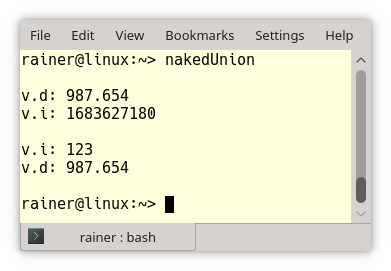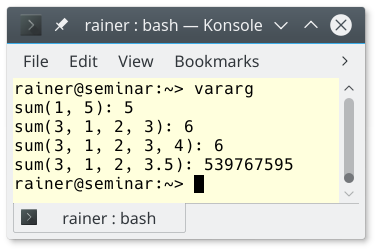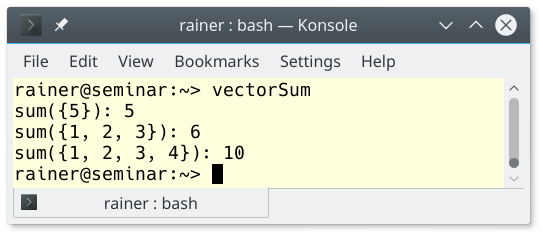# C++ Core Guidelines: Type Safety by Design

What does that mean: type safety by design. Type safety by design just means that you always initialize your variables, use std::variant instead of a union, or prefer variadic templates and fold expressions to va_arg‘s.As in my first post to type safety C++ Core Guidelines: Type Safety, I will name the four missing types of type safety and add additional information, if necessary. Here we are:

## Type Safety

### Type.5: Don’t use a variable before it has been initialized

The rules on which object will be initialized are quite difficult to get in C++. Here is a simple example.

```struct T1 {};
class T2{
public:
T2() {}
};

int n;                 // OK

int main(){
int n2;              // ERROR
std::string s;       // OK
T1 t1;               // OK
T2 t2;               // OK
}
```

n is a global variable; therefore, it is initialized to 0. This initialization will not hold for n2 because it is a local variable and is, therefore, not initialized. But they are initialized if you use a user-defined type such as std::string, T1, or T2 in a global or local scope.

If that is too difficult for you, I have a simple fix. Use auto. The c ompiler can not guess from an expression auto a what type a has to be. Now, you can not forget to initialize the variable. You are forced to initialize your variables.

##Modernes C++ Mentoring

Be part of my mentoring programs:

• "Fundamentals for C++ Professionals" (open)
• "Design Patterns and Architectural Patterns with C++" (open)
• "C++20: Get the Details" (reopens December 2023)
• Do you want to stay informed about my mentoring programs: Subscribe via E-Mail.

```struct T1 {};
class T2{
public:
T2() {}
};

auto n = 0;

int main(){
auto n2 = 0;
auto s = ""s;
auto t1 = T1();
auto t2 = T2();
}
```

### Type.6: Always initialize a member variable

In this case, I can make it short. I have already written in my post C++ Core Guidelines: More Non-Rules and Myths, about the initialization of member variables.

### Type.7: Avoid naked union

First of all: What is a union? A union is a user-defined type that can hold one of its members at a time.

“Naked” unions are error-prone because you must keep track of the underlying type.

```// nakedUnion.cpp

#include <iostream>

union Value {
int i;
double d;
};

int main(){

std::cout << std::endl;

Value v;
v.d = 987.654;  // v holds a double
std::cout << "v.d: " << v.d << std::endl;
std::cout << "v.i: " << v.i << std::endl;      // (1)

std::cout << std::endl;

v.i = 123;     // v holds an int
std::cout << "v.i: " << v.i << std::endl;
std::cout << "v.d: " << v.d << std::endl;      // (2)

std::cout << std::endl;

}
```

The union holds int the first iteration a double and in the second iteration an int value. You get undefined behavior if you read a double as an int (1) or an int as a double (2).### std::variant

A std::variant is, in contrast, a type-safe union. We have had it since C++17. An instance of std::variant has a value from one of its types. The type must not be a reference, array, or void. A default-initialized std::variant will be initialized with its first type. In this case, the first type must have a default constructor. Here is a simple example based on cppreference.com.

```// variant.cpp

#include <variant>
#include <string>

int main(){

std::variant<int, float> v, w;       // (1)
v = 12;
int i = std::get<int>(v);
w = std::get<int>(v);                // (2)
w = std::get<0>(v);                  // (2)
w = v;                               // (2)

//  std::get<double>(v);   // error: no double in [int, float] (3)
//  std::get<3>(v);        // error: valid index values are 0 and 1 (4)

try{
std::get<float>(w);                // (5)
}

std::variant<std::string> v2("abc"); // (6)
v2 = "def";                          // (7)

}
```

I define in line (1) both variants v and w. Both can have an int and a float value. std::get<int>(v) returns the value. In line (2), you see three possibilities to assign the variant v to the variant w. But you have to keep a few rules in mind. You can ask for the value of a variant by type (line 3) or index (line 4). The type must be unique and the index valid. On line (5), the variant w holds an int value. Therefore, I get a std::bad_variant_access exception. A conversion can occur if the constructor call or assignment call is unambiguous. That is the reason that it’s possible to construct a std::variant<std::string> in line (6) with a C-string or assign a new C-string to the variant (line 7).

### Type.8: Avoid varargs

Variadic functions include std::printf which can take an arbitrary number of arguments. The issue is that you must assume that the correct types were passed. Of course, this assumption is very error-prone and relies on the programmer’s discipline.

Here is a small example to understand the implicit danger of variadic functions.

```// vararg.cpp

#include <iostream>
#include <cstdarg>

int sum(int num, ... ){

int sum{};

va_list argPointer;
va_start(argPointer, num );
for( int i = 0; i < num; i++ )
sum += va_arg(argPointer, int );
va_end(argPointer);

return sum;
}

int main(){

std::cout << "sum(1, 5): " << sum(1, 5) << std::endl;
std::cout << "sum(3, 1, 2, 3): " << sum(3, 1, 2, 3) << std::endl;
std::cout << "sum(3, 1, 2, 3, 4): " << sum(3, 1, 2, 3, 4)  << std::endl; // (1)
std::cout << "sum(3, 1, 2, 3.5): " << sum(3, 1, 2, 3.5) << std::endl;    // (2)

}
```

sum is a variadic function. Its first argument is the number of arguments that should be summed up. I will only provide so much info to the varargs macros so you can understand the program. For more information, read cppreference.com.

• va_list: holds the necessary information for the following macros
• va_arg: accesses the next variadic function argument
• va_end: end the access of the variadic function arguments

In line (1) and line (2), I had a bad day. First, the number of arguments is wrong; second, I provided a double instead of an int. The output shows both issues. The last element in line (1) is missing, and the double is interpreted as int (line 2).This issue can be easily overcome with fold expressions in C++17:

```// foldExpressions.cpp

#include <iostream>

template<class ...Args>
auto sum(Args... args) {
return (... + args);
}

int main(){

std::cout << "sum(5): " << sum(5) << std::endl;
std::cout << "sum(1, 2, 3): " << sum(1, 2, 3) << std::endl;
std::cout << "sum(1, 2, 3, 4): " << sum(1, 2, 3, 4)  << std::endl;
std::cout << "sum(1, 2, 3.5): " << sum(1, 2, 3.5) << std::endl;

}
```

Okay, the function sum may look terrifying to you. C++11 supports variadic templates. These are templates that can accept an arbitrary number of arguments. A parameter pack holds the arbitrary number denote by an ellipse (…). Additionally, with C++17, you can directly reduce a parameter pack with a binary operator. This addition, based on variadic templates, is called fold expressions. In the case of the sum function, the binary + operator (…+ args) is applied. If you want to know more about fold expressions in C++17, here is my previous post on it.

The output of the program is as expected:Additionally to variadic templates and fold expression, there is another comfortable way for a function to accept an arbitrary number of arguments of a specific type: use a container of the STL such as std::vector as an argument.

`// vectorSum.cpp`

#include <iostream>
#include <numeric>
#include <vector>

auto sum(std::vector<int> myVec){
return std::accumulate(myVec.begin(), myVec.end(), 0);
}

int main(){

std::cout << “sum({5}): “ << sum({5}) << std::endl;
std::cout << “sum({1, 2, 3}): “ << sum({1, 2, 3}) << std::endl;
std::cout << “sum({1, 2, 3, 4}): “ << sum({1, 2, 3, 4}) << std::endl;

}

In this case, a std::initializer_list<int> is used as an argument of the function sum. A std::initializer_list can directly initialize a std::vector. In contrast, to fold expressions, is performed at runtime.## What’s next?

Next time, I will continue with the profile to Bounds Safety. This profile has four rules.

Thanks a lot to my Patreon Supporters: Matt Braun, Roman Postanciuc, Tobias Zindl, G Prvulovic, Reinhold Dröge, Abernitzke, Frank Grimm, Sakib, Broeserl, António Pina, Sergey Agafyin, Андрей Бурмистров, Jake, GS, Lawton Shoemake, Jozo Leko, John Breland, Venkat Nandam, Jose Francisco, Douglas Tinkham, Kuchlong Kuchlong, Robert Blanch, Truels Wissneth, Kris Kafka, Mario Luoni, Friedrich Huber, lennonli, Pramod Tikare Muralidhara, Peter Ware, Daniel Hufschläger, Alessandro Pezzato, Bob Perry, Satish Vangipuram, Andi Ireland, Richard Ohnemus, Michael Dunsky, Leo Goodstadt, John Wiederhirn, Yacob Cohen-Arazi, Florian Tischler, Robin Furness, Michael Young, Holger Detering, Bernd Mühlhaus, Matthieu Bolt, Stephen Kelley, Kyle Dean, Tusar Palauri, Dmitry Farberov, Juan Dent, George Liao, Daniel Ceperley, Jon T Hess, Stephen Totten, Wolfgang Fütterer, Matthias Grün, Phillip Diekmann, Ben Atakora, Ann Shatoff, Rob North, Bhavith C Achar, and Marco Parri Empoli.

Thanks, in particular, to Jon Hess, Lakshman, Christian Wittenhorst, Sherhy Pyton, Dendi Suhubdy, Sudhakar Belagurusamy, Richard Sargeant, Rusty Fleming, John Nebel, Mipko, Alicja Kaminska, Slavko Radman, and David Poole.

 My special thanks to EmbarcaderoMy special thanks to PVS-StudioMy special thanks to Tipi.buildMy special thanks to Take Up Code## Seminars

I’m happy to give online seminars or face-to-face seminars worldwide. Please call me if you have any questions.

### Standard Seminars (English/German)

Here is a compilation of my standard seminars. These seminars are only meant to give you a first orientation.

• C++ – The Core Language
• C++ – The Standard Library
• C++ – Compact
• C++11 and C++14
• Concurrency with Modern C++
• Design Pattern and Architectural Pattern with C++
• Embedded Programming with Modern C++
• Generic Programming (Templates) with C++

#### New

• Clean Code with Modern C++
• C++20

### Contact Me

Modernes C++ Mentoring,Tags:
0 replies